## Wednesday, January 15, 2014

### Arduino - Improving refresh rate of a TFT LCD display

I'm working on a digital speedometer project for my car and I ordered a couple different displays from Adafruit to experiment with. One of the displays I ordered is a 2.2" color TFT LCD display. I was really hoping to use this display to show the current speed of my car. One concern I had about using it for that purpose was the speed that an Arduino board could refresh the information on the screen. To test the display I wrote a simple sketch that just displays the number of seconds since start. Here is a video of that first attempt:

The redraw time causes the numbers to look like they are flashing which is very annoying. The mistake I made was blanking out the entire screen with tft.fillScreen(ILI9340_BLACK). It takes quite a while to redraw the entire screen with black.

Next I tried drawing a black rectangle just on the area of the screen where I was drawing numbers. Here is the result:

Huge improvement. Only blanking out that small area greatly sped up the redraw time.
In this next video I have highlighted the redraw area in red to make it easier to see what I am talking about:

What I am realizing at this point is you should only redraw information that has changed. If you watch the last video again, once the number goes past single digits the left digit looks like it is flashing. This is because it is being blanked out and redrawn every time the number changes. When you redraw something that hasn't changed it looks like flashing. Well that's it for now. I am going to continue to work on this more and figure out how to selectively redraw only digits that change. Once I figure that out I'll write up another blog post.
[UPDATE] - Here is part two:
http://matthewcmcmillan.blogspot.com/2014/08/arduino-tft-lcd-display-refresh-rate-part2.html

Here is the code I was using to do my testing.

1.You could try writing over the text with the old text, but in black.

int old_millis;
tft.setCursor(120, 70);
tft.setTextColor(ILI_9340_BLACK);
tft.print(old_millis);
tft.setCursor(120, 70);
tft.setTextColor(ILI_9340_WHITE);
old_millis = millis()/1000;
tft.print(old_millis);

1.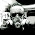That is exactly what I ended up doing. Another technique I used was to only update digits that have changed. This video shows the results of that:

2.I wrote some code to display numbers as seven segment digits and draw the numbers with only seven calls to fillRectangle. It was the only way to update the display fast enough to show tenths of a second updates. It uses both a foreground and background color so you don't have to clear the space each time and can blank leading zeros. The size array is documented so you can create more sizes if needed. Maybe this will help others who need faster numbers.

[code]
//Draws a Seven Segment Number starting at location xoff/yoff with forground color Color and and background color BColor
//By William Zaggle
void drawNumber(int number,int xLoc,int yLoc,int cSize, int fColor, int bColor, char nDigits)
{
unsigned char numbers= {0x3F,0x06,0x5B,0x4F,0x66,0x6D,0x7D,0x07,0x7F,0x67,0x00}; // seven segment number definitions 0-9 plus blank 10
// siz defines the size of number where
// 0 = width of top,center & bottom segments
// 1 = height of top, center & bottom segments and width of side segments
// 2 = top offset of side segments (top center segment always offset 0)
// 3 = height fo side segments (typically taller than center segments are wide)
// 4 = height offset of lower side segments
// 5 = height offset of lower center segment
// 6 = height offset of middle center segment
// 7 = width offset of center segments
// 8 = width offset of right side segments (left side segments always offset 0)
// 9 = width of number including right side space between numbers
unsigned char siz = {{3,1,1,5,7,12,6,2,6,11},{6,2,1,10,13,22,10,3,10,17},{8,3,1,13,16,27,14,5,15,23},{10,3,1,15,18,31,15,5,17,25},{12,4,2,17,21,35,18,6,20,29},{15,5,2,20,24,41,21,7,23,35}};
int i=0,j=1,n=1;
char cnt = abs(nDigits); //cnt = number of digits wanted in display
for (i=number;i>9;i/=10,++j) n*=10; //j = number of digits needed to display and n = 10 to the power of j-1
for (;j9;i/=10,++j) n*=10; //j = number of digits needed to display and n = 10 to the power of j-1
for (;j<cnt;++j) n*=10; //bump n & j to meet cnt if smaller (if larger then only most significant cnt numbers are shown)
while (cnt) {
i = int(number/n); // i = number to show
number -= (i*n); // remove i from the front of the number
n = n/10; // prepare next power of 10
--cnt;
if ((nDigits<0) && (i==0)) i = 10; // show blank in place of leading zero if Digits < 0;
// Starting X Y W H Mask
// ------------------ ----------------- ------------- ------------- --------------------
Tft.fillRectangle(siz[cSize]+xLoc, yLoc, siz[cSize], siz[cSize], (numbers[i] & 1)?fColor:bColor); // top
Tft.fillRectangle(siz[cSize]+xLoc, siz[cSize]+yLoc, siz[cSize], siz[cSize], (numbers[i] & 2)?fColor:bColor); // top right
Tft.fillRectangle(siz[cSize]+xLoc, siz[cSize]+yLoc, siz[cSize], siz[cSize], (numbers[i] & 4)?fColor:bColor); // bottom right
Tft.fillRectangle(siz[cSize]+xLoc, siz[cSize]+yLoc, siz[cSize], siz[cSize], (numbers[i] & 8)?fColor:bColor); // bottom
Tft.fillRectangle( xLoc, siz[cSize]+yLoc, siz[cSize], siz[cSize], (numbers[i] & 16)?fColor:bColor); // bottom left
Tft.fillRectangle( xLoc, siz[cSize]+yLoc, siz[cSize], siz[cSize], (numbers[i] & 32)?fColor:bColor); // top left
Tft.fillRectangle(siz[cSize]+xLoc, siz[cSize]+yLoc, siz[cSize], siz[cSize], (numbers[i] & 64)?fColor:bColor); // middle
xLoc += siz[cSize]; //space for next number
}
}

2.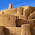Hi, I having been having trouble doing same thing and I was hoping if you could share the code use in updating the digits that changed.

Thanks

1.I have finished the follow up post to this one. The code is in this post.

http://matthewcmcmillan.blogspot.com/2014/08/arduino-tft-lcd-display-refresh-rate-part2.html

3.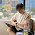Thanks! This is a good tip!

4.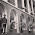Hi Matt

I have Adafruit 2.2 TFT LCD, now I'm working on my final project, and I have problems in access this LCD. I want to display the sensor readings with the background of each, there are several kinds of sensors with each background. The problem is, how to display the sensor readings with each background in the 'void loop' but not repeat as the 'void setup' (only called once). In my system there are several sub-menus, and each submenu that I want the background is not repeated (like refresh) but still be able to perform the measurement sensor. I hope you can help me...

thank you

1.If you can share your code with me it would be much easier to try and understand you problem. Can you post it on gist.github.com?

5.I'm sorry but all this work is useless.
You can use the overloaded function tft.setTextColor(ILI_9340_WHITE, ILI_9340_BLACK) where the first parameter is the color of the text, and the second one is the color of the background. Try it :)

1.That is it, right on.

6.Here is a better version than I posted earlier, and might make your numbers look nicer and will still display fast enough to show real time change in speed. Plus with both a foreground and background color option they clear themselves as they change. There is also a link to where I posted it on the Arduino Forum.

http://forum.arduino.cc/index.php?topic=307348.0

/**********************************************************************************
Routine to Draw Large 7-Segment formated number with Arduino TFT Library
by William Zaggle (Uses TFT Library DrawLine functions).

int n - The number to be displayed
int xLoc = The x location of the upper left corner of the number
int yLoc = The y location of the upper left corner of the number
int cSe = The size of the number. Range 1 to 10 uses Large Shaped Segments.
fC is the foreground color of the number
bC is the background color of the number (prevents having to clear previous space)
nD is the number of digit spaces to occupy (must include space for minus sign for numbers < 0)
nD < 0 Suppresses leading zero

Sample Use: Fill the screen with a 2-digit number showing current second suppressing leading zero

draw7Number(second(),20,40,10,WHITE, BLACK, -2);

**********************************************************************************/
void draw7Number(int n, unsigned int xLoc, unsigned int yLoc, char cS, unsigned int fC, unsigned int bC, char nD) {
unsigned int num=abs(n),i,s,t,w,col,h,a,b,si=0,j=1,d=0,S1=cS,S2=5*cS,S3=2*cS,S4=7*cS,x1=(S3/2)+1,x2=(2*S1)+S2+1,y1=yLoc+x1,y3=yLoc+(2*S1)+S4+1;
unsigned int seg={{(S3/2)+1,yLoc,1},{x2,y1,0},{x2,y3+x1,0},{x1,(2*y3)-yLoc,1},{0,y3+x1,0},{0,y1,0},{x1,y3,1}};
unsigned char nums={0x3F,0x06,0x5B,0x4F,0x66,0x6D,0x7D,0x07,0x7F,0x67,0x00,0x40},c=(c=abs(cS))>10?10:(c<1)?1:c,cnt=(cnt=abs(nD))>10?10:(cnt<1)?1:cnt;
for (xLoc+=cnt*(d=(2*S1)+S2+(2*S3)+2);cnt>0;cnt--){
for (i=(num>9)?num%10:((!cnt)&&(n<0))?11:((nD<0)&&(!num))?10:num,xLoc-=d,num/=10,j=0;j<7;++j){
col=(nums[i]&(1<<j))?fC:bC;s=(2*S1)/S3;
if (seg[j])for(w=S2,t=seg[j]+S3,h=seg[j]+(S3/2),a=xLoc+seg[j]+S1,b=seg[j];b<h;b++,a-=s,w+=(2*s))Tft.drawHorizontalLine(a,b,w,col);
else for(w=S4,t=xLoc+seg[j]+S3,h=xLoc+seg[j]+S3/2,b=xLoc+seg[j],a=seg[j]+S1;b<h;b++,a-=s,w+=(2*s))Tft.drawVerticalLine(b,a,w,col);
for (;b<t;b++,a+=s,w-=(2*s))seg[j]?Tft.drawHorizontalLine(a,b,w,col):Tft.drawVerticalLine(b,a,w,col);
}
}
}

1.Thanks for contributing William! This is great. I'll have to try it out soon.

2.William, I finally got around to trying out your code for drawing numbers. Works great!

7.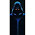Im back at it lol.
Serious suspension issues i had to focus on. Any how first, i must stress, I'm not here "demanding help" so to speak, nor here to correct you in any way. You, are the only person i know, even online, with vss experience, so, here i am.

Got everything lined up, got a signal
But, speed reports are very sketchy. Sometimes, over 250mph. Seems to only be stable in the 30s. Ford vic. 8000 pulses (from reports online)

So, what do you think may be the issue there?

8.9.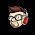can i make this project with uno and tft mcufriends shield?
because i got same problem with the tft

Please note all comments are moderated by me before they appear on the site. It may take a day or so for me to get to them. Thanks for your feedback.